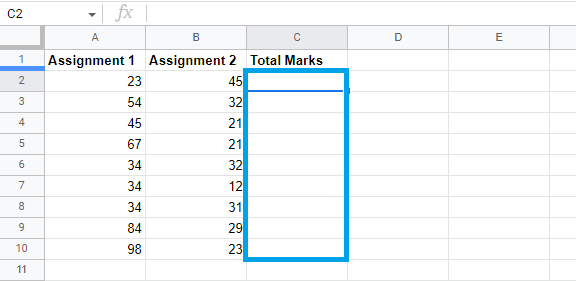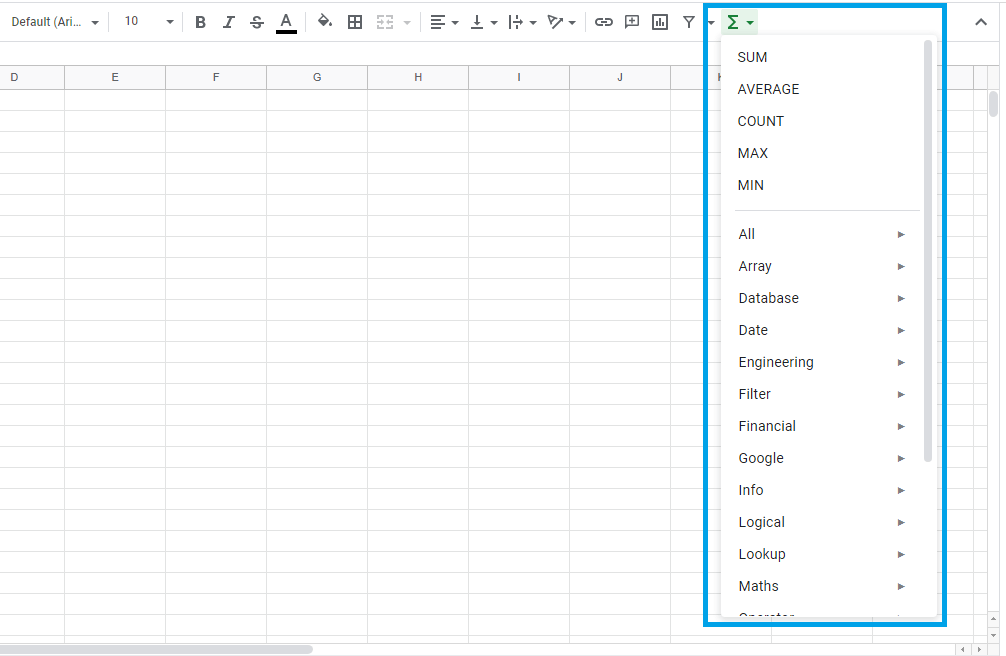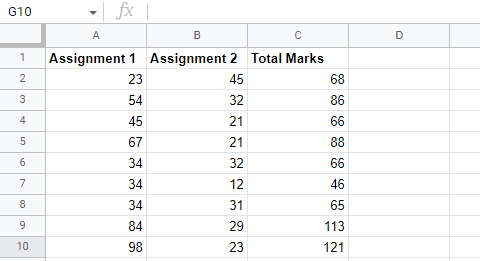# How to create formulas using functions in Google Sheets

Published June 12, 2022

With Google Sheets' "functions" feature, you can create formulas using a number of different functions. This feature allows you to solve different mathematical problems quickly and easily. In this article, we are going to learn how to create formulas using functions in Google Sheets. We will also learn the syntax and use of functions in Google Sheets. Let's get started.

## An Introduction to Google Sheets Functions

When writing functions, just like formulae in Google Sheets, you must follow a specified sequence called syntax. To build a formula in a function in Google Sheets, enter an equals sign (=), the name of the function, and finally the argument. The data to be computed by the formula is often contained in the arguments.

Example: =SUM (C2:C10)

Depending on the syntax for the function, arguments can contain either cell ranges or individual cells enclosed in parentheses. If more than one argument is needed, you can include it. As an example, to add cells within a range, we can use =SUM (C1:C3), which will add cells between C1 and C3.

A function that takes more than one argument is usually separated by a comma. For example, we can use the SUM function with several arguments, =SUM (C1:C3, C5: C8, D1:D7)

## How to create a function in Google Sheets

Creating a function in Google Sheets is quite easy.  To do so, just follow the following simple steps:

Step 1: The first step is to select a mathematical function that corresponds to the mathematical function that you wish to perform. While Google Sheets provides quite a few common mathematical functions, here is a list of the most common ones.

• AVERAGE: By using this function, you can calculate the average of the values specified by arguments.

• COUNT: When you are using Count, you can count the number of cells in an argument with numerical data.

• MAX: In this case, the MAX function gives you the highest value within the range of selected cells in the argument.

• MIN: By using this function, you will be able to find the lowest value that exists within the cells included in an argument.

• SUM: By using this function, you can add the values of cells in an argument.

Step 2: Having decided on the function to use, we now need to specify the range of cells to be included in our function argument. In our case, we are going to work with cells C2 to C10.Step 3: Next, click on the Functions symbol in your Google Sheets menu, and from the drop-down menu, pick the formula that you want to use.In our case, we are going to work with the SUM function.Step 4: Enter the cell references to add after selecting the function to use. In this case, we are going to use A2: B2Step 6: Click on the cell fill handle and drag the handle down the cells to select the cells you want the function calculated on. In this case, we want to drag the handle from C2 to C10.Step 7: Upon releasing the mouse, the function is calculated and the results are displayed in the cells C2 to C10.Conclusion

Lastly, it is important to note that Google Sheets will not tell you if you have errors in your function. As a result, you will need to check whether all the functions were generated correctly in all selected cells. To do so, double-click on the cells and check the functions of the formula

How do i create new google sheet

How to delete a sheet in google sheet

How to modify rows width columns and cells in google sheet

How to copy and paste cells in google sheet

How to drag and drop cells in google sheet

How to insert data using the fill handle the feature google sheet

How to insert,move and delete rows and columns google sheet

How to select cells in google sheet

How to freeze rows and columns google sheet

How to wrap text and merge cells google sheet

How to change the font size in google sheet

How to change the font in google sheet

How to change text color in google sheet

How to make text bold in google sheet

How to change the cell background color google sheet

How do i align text in google sheet

How to create a complex formula using the orderof operations google sheet

How to create and copy formulas using relative references google sheet

How to use absolute references to create a copy and paste formula google sheet

create formulas using functions in google sheets

How to sort and filter data in google sheets

How to insert image in google sheets

How to rotate text in google sheets

How to enable spell checker in google sheets

How to enable auto complete in google sheets

 Article Contributed By :116 Views